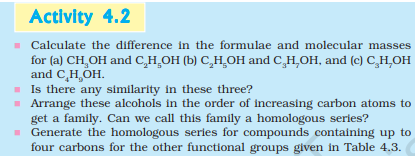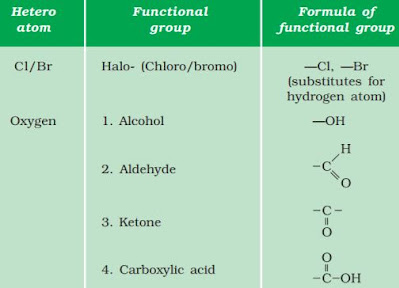Home » Class 10 » Class 10 Science » Activity 4.2 for Class 10 Science – Carbon and its compounds

# Activity 4.2 for Class 10 Science – Carbon and its compounds

## Activity 4.2 NCERT Class 10 Science: Understanding Homologous Series in Organic Chemistry

For students seeking solutions to Activity 4.2 for Class 10 Science in NCERT, you’ve come to the right spot. This article provides an explanation and conclusion for NCERT Science Class 10 Chapter 4 Activity.### Aim of the Activity

The aim of this activity is to help students understand

• the concept of a homologous series in organic chemistry,
• identify the consistent pattern in molecular masses within the series, and
• apply this understanding to generate new homologous series for other functional groups.

### Discussion on Activity

Let’s get into the details by talking about the molecular masses of Methanol ($CH_3OH$), Ethanol ($C_2H_5OH$), Propanol ($C_3H_7OH$), and Butanol ($C_4H_9OH$). The molecular masses of these compounds are computed as follows:

Methanol ($CH_3OH$)

$CH_3OH = 12.01$ (for $C$) $+ 3 \times 1.01$ (for $H$) $+ 16$ (for $O$) $+ 1.01$ (for $H$) $= 32.05$ u

Ethanol ($C_2H_5OH$)

$C_2H_5OH = 2 \times 12.01$ (for $C$) $+ 5 \times 1.01$ (for $H$) $+ 16$ (for $O$) $+ 1.01$ (for $H$) $= 46.07$ u

Propanol ($C_3H_7OH$)

$C_3H_7OH = 3 \times 12.01$ (for $C$) $+ 7 \times 1.01$ (for $H$) $+ 16$ (for $O$) $+ 1.01$ (for $H$) $= 60.09$ u

Butanol ($C_4H_9OH$)

$C_4H_9OH = 4 \times 12.01$ (for $C$) $+ 9 \times 1.01$ (for $H$) $+ 16$ (for $O$) $+ 1.01$ (for $H$) $= 74.12$ u

This table shows the molecular masses of each pair of compounds, and the difference in molecular mass between them, which corresponds to the mass of a $CH_2$ group (14.02 u), confirming that they belong to the same homologous series.

Pair of Compounds Molecular Mass of First Compound (u) Molecular Mass of Second Compound (u) The difference in Molecular Mass (u)
$CH_3OH$ and $C_2H_5OH$ 32.05 46.07 14.02
$C_2H_5OH$ and $C_3H_7OH$ 46.07 60.09 14.02
$C_3H_7OH$ and $C_4H_9OH$ 60.09 74.12 14.03

### Observations and Their Explanation

#### Differences in Molecular Masses

The consistent difference in molecular masses among successive compounds in a series is due to the addition of a $CH_2$ group.

#### Chemical Structure Similarity

Each subsequent molecule in the series has an additional $CH_2$ group, indicating that these compounds are part of a homologous series.

#### Existence of Homologous Series

By arranging the compounds in order of increasing carbon atoms, they form a homologous series. This series is characterized by a constant difference in molecular formula, which in this case is a $CH_2$ group.

#### Extension to Other Functional Groups

The pattern of a consistent difference (one $CH_2$ group) is not exclusive to alcohols but can be extended to other functional groups. This shows the universal feature of homologous series in organic chemistry. Each successive member in a homologous series can be obtained by adding a $CH_2$ group to the previous member.

### Solutions to Questions in Activity 4.2

#### Question 1

Calculate the differences in the formulae and molecular masses for the following pairs of compounds: $CH_3OH$ and $C_2H_5OH$, $C_2H_5OH$ and $C_3H_7OH$, $C_3H_7OH$ and $C_4H_9OH$.

For each pair, the difference in the formula is a $-CH_2-$ group. This results in a difference in molecular mass of 14 units (u).

#### Question 2

Is there any similarity in these three?

Yes, there is a significant similarity among these three sets of compounds. In each case, the difference in molecular mass between the two compounds is approximately $14.02$ u.

This difference corresponds to the mass of a $CH_2$ group, indicating that each subsequent molecule in the series has one more $CH_2$ group than the previous one. Thus, the primary similarity is that they all belong to a homologous series.

#### Question 3

Arrange these alcohols in the order of increasing carbon atoms to get a family. Can we call this family a homologous series?

The given alcohols arranged in the order of increasing number of carbon atoms are: Methanol ($CH_3OH$), Ethanol ($C_2H_5OH$), Propanol ($C_3H_7OH$), and Butanol ($C_4H_9OH$).

Yes, we can call this family a homologous series. A homologous series is a series of organic compounds having the same functional group and similar chemical properties due to the presence of the same functional group. In each subsequent member, the molecular mass differs by a $CH_2$ group ($14.02$ u).

#### Question 4

Generate the homologous series for compounds containing up to four carbons for the other functional groups given in Table 4.3 given below.• Chloro group: $CH_3Cl$, $C_2H_5Cl$, $C_3H_7Cl$, $C_4H_9Cl$
• Aldehyde group: $CH_3CHO$, $C_2H_5CHO$, $C_3H_7CHO$, $C_4H_9CHO$
• Ketone group: $CH_3COCH_3$, $C_2H_5COCH_3$, $C_3H_7COCH_3$, $C_4H_9COCH_3$
• Carboxylic acid group: $CH_3COOH$, $C_2H_5COOH$, $C_3H_7COOH$, $C_4H_9COOH$